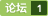### 如何将double转换成int? [问题点数：10分，结帖人Sco_field]JAVA中doubleint类型按四舍五入取整(实用)
public static void main(String[] args) { System.out.pr<em>int</em>ln(&quot;向上取整:&quot; + (<em>int</em>) Math.ceil(96.1));// 97 (去掉小数凑整:不管小数是多少，都进一) System.out.pr<em>int</em>ln(&quot;向下取整&quot; + (<em>int</em>) Math.floor(96.8));// 96 (去掉小数...
intdouble

C++中如何把double类型转化为int?
RT 在线等
C语言的int, float,double相互转化（从本质上理解可能的问题）

doubleint 的问题
-

double类型相加精度问题解决
<em>double</em> Sum = Add(Convert.ToDouble(values.GetValue(85, i)), Convert.ToDouble(values.GetValue(86, i)));      #region 加         private <em>double</em> Add(<em>double</em> add1, <em>double</em> add2)         {             do

<em>double</em> v = 52428.8; var v1 = v * 2; var v2 = v * 3; Console.WriteLine(v1); Console.WriteLine(v2); C
double型强转int型--向下取整；+0.5实现四舍五入
public class test2 { public static void main(String[] args) { // TODO Auto-generated method stub <em>double</em> a = 100.1; <em>double</em> b = 100.5; float c = 100.1f; float d = 100.5f; System.out.pr<em>int</em>l
double类型转int类型，精度丢失的问题

doubleint的快速转换
<em>double</em>到<em>int</em>的快速转换  (2014-01-19 13:39:56) 转载▼ 标签：  <em>double</em>   long   数值类型转换   快速   图形表示用<em>double</em>，显示用<em>int</em>，很常规的提高效率的小方子。可是，你知道吗？最普通的类型转换其实是相当慢的: <em>int</em> a = (<em>int</em>)dbl; 今
java中向上向下及四舍五入取整的方法，double型强制转换成int型的取整方法？
java 向上或向下以及四舍五入取整的方法，Java 中<em>double</em>型强制<em>转换成</em><em>int</em>型时是怎么取整的？向上取整：Math.ceil(<em>double</em> a) 向下取整：Math.floor(<em>double</em> a) 四舍五入取整：Math.r<em>int</em>(<em>double</em> a) 例子1（三种取整的方法）： <em>double</em> a=35; <em>double</em> b=20; <em>double</em> c = a/b; //等于1.7
java中doubleint
java中<em>double</em>转<em>int</em>    (1)、 (<em>int</em>) 2.9 = 2;//不进行四舍五入    (2)、  四舍五入：new BigDecimal("1.5").setScale(0, BigDecimal.ROUND_HALF_EVEN)；  其中half_even: 2.5=2; 1.5=2; （类似于银行家算法）        half_up: 2.5=3, 1.5=2;
java中将double转型为int。java中的幕运算方法Math.pow()。
java中将<em>double</em>转型为<em>int</em>有2个方法。 1： <em>double</em> d = 12.0; <em>int</em> i = (new Double(d)).<em>int</em>Value(); 2： <em>double</em> d = 12.0; <em>int</em> i = (<em>int</em>)d; 不要使用(<em>int</em>)来强制转换  应该先生成一个<em>double</em>的外覆型D
java string转double再转int 四舍五入

java把含小数点的数字字符串转换为int类型
String num =&quot;1.00&quot;;<em>int</em> abc =Double.valueOf(num).<em>int</em>Value();//转换为Int类型
java---doubleint的问题

Java类型转换: intdouble

Java中 float、double使用注意问题（转载）

float转int的问题，跪求大神解释？
-
java中Object转换成int或String类型方法
String转换为<em>int</em>类型的方法： 1. Integer.parseInt([String]) 2.Integer.valueOf([String]).<em>int</em>Value(); 3.Integer.decode([String]):将 String 解码为 Integer。接受通过以下语法给出的十进制、十六进制和八进制数字 例如： <em>int</em> a=Integer.decode("0144"); //八...
java 中把double类型转成 int类型
<em>double</em> d = 1.233; String s1 = String.valueOf(d); String s2 = s1.substring(0, s1.indexOf(".")) + s1.substring(s1.indexOf(".")+1); <em>int</em> i = Integer.parseInt(s2);

-
Java中double类型数字转换（整数时候，去掉小数点，小数时候，保留两位小数）
/* * 如果是小数，保留两位，非小数，保留整数 * @param number */ public static String getDoubleString(<em>double</em> number) { String numberStr; if (((<em>int</em>) number * 1000) == (<em>int</em>) (number ...
Java中String类型，int类型，double类型相互转换
1、整数<em>int</em>转成字符串String//<em>int</em>转成String <em>int</em> i = 123456; String s1 = String.valueOf(i); //方法一 String s2 = Integer.toString(i); //方法二 String s3 = ""+i; //方法三 System.out.pr<em>int</em>l
java 将 Double类型的数据转换为Int 类型 四舍五入
package util; import java.text.DecimalFormat; public class test { public static void main(String[] args) { DecimalFormat df = new DecimalFormat("######0"); //四色五入<em>转换成</em>整数 Double x=3.14159; Do
Java中double型转化成int
doupower = (sum / 10.0 * m_iLevel) / 10000; // str += String.format("%.1f", power); // java中<em>double</em>型转化成<em>int</em>型,进行四舍五入操作： tmp = Integer.parseInt(new java.text.DecimalFormat("0")                       .
Java的文件读写操作
file(内存)----输入流--【程序】----输出流--file(内存) 当我们读写文本文件的时候，采用Reader是非常方便的，比如FileReader，InputStreamReader和BufferedReader。其中最重要的类是InputStreamReader， 它是字节转换为字符的桥梁。你可以在构造器重指定编码的方式，如果不指定的话将采用底层操作系统的默认编
java中基本数据类型之间的转换 int double byte short

Java 中Double型和double的取整:intValue是去尾取整,负的向上，正的向下，Math.floor才是真的向下取整
public class Double2Int { /** * @param args */ public static void main(String[] args) { // TODO Auto-generated method stub <em>double</em> d = 5.5; <em>int</em> i = (<em>int</em>)d;
java各类型互转
<em>如何将</em>字串 String <em>转换成</em>整数 <em>int</em>?  <em>int</em> i = Integer.valueOf(my_str).<em>int</em>Value(); <em>int</em> i=Integer.parseInt(str); <em>如何将</em>字串 String <em>转换成</em>Integer ? Integer
sqlserver将int类型转为double类型的问题

java中int, double 转化成String, String转化成double,怎么做?请给个例子.
-
int 怎么转换double求大神指点
-
js实现intdouble的类型转换
js实现<em>int</em>到<em>double</em>的类型转换 如：把3<em>转换成</em>3.00。 我们知道可以自己判断并添加.00，但不希望这样做，希望有类似java的直接转换的方法。
int、char、double与byte类型之间相互转换
<em>int</em>、char、<em>double</em>与byte相互转换
Java字符串转doubleint

java中忽略字母大小写判断相等
String a="ABCD"; String b="abcd"; System.out.pr<em>int</em>ln(a.equalsIgnoreCase(b)); 结果输出为true
string去除重复字符两个方法
string去除重复字符两个方法 网上有很多伪方法，只删除了相邻重复字符串，大家警惕！ 主要使用list.contains()检测重复。 一直在想本来应该怎样做，因为面试官说了句只用了一层循环，可能这样是比较通用的： 1、charAt遍历String每个字符（第一层循环） 2、charAt遍历StringBuffer每个字符，...

https://www.imooc.com/qadetail/168807
C++中intdouble与string的相互转换

C++、C中有关double、float类型转换成int型，及取整，丢失精度问题。

c语言double类型printf问题

double强转换为int，除了静度丢失还有什么状况？
<em>double</em>是64位，<em>int</em>是32位，<em>int</em>最大值为2^31-1=2147483647。所以当<em>double</em>大于2147483647时，强转为<em>int</em>型时值为  2147483647 *****有何问题，还望大神指出*****
float 和int转换是怎么做到的

Java把含小数点的数字字符串转换为int类型
Java把含小数点的数字字符串（例1.01）转换为<em>int</em>类型 String num =“1.01”; <em>int</em> abc =Double.valueOf(num).<em>int</em>Value();//转换为Int类型
doubleint精度丢失
<em>double</em> x; <em>int</em> y; x=0.03; y=(<em>int</em>)(x*100); y成2了，我想要的是3啊，这是怎么回事，应该怎么搞法？

C++中将string类型转换为int, float, double类型 主要通过以下几种方式：
C++中将string类型转换为<em>int</em>, float, <em>double</em>类型 主要通过以下几种方式： # 方法一： 使用stringstream stringstream在<em>int</em>或float类型转换为string类型的方法中已经介绍过， 这里也能用作将string类型转换为常用的数值类型。 Demo: #include #include //使用stringstream需要引入这个头文件
int类型转为double

vs2015错误代码c4244:从“double”转换到“int”，可能数据丢失。
vs2015错误代码c4244:从“<em>double</em>”转换到“<em>int</em>”，可能数据丢失。解决方法如下：1，主函数名“main”抒写错误，即写成“mian，man”等；2，这是警告，可以去掉4244警告或者就是强制转换”<em>double</em>”到“<em>int</em>”；3，<em>int</em> is_prime（n）改成<em>int</em> is_prime（<em>int</em> n）。...

doubleint精度丢失问题

java从Object类型转换成double,int类型
import java.math.BigDecimal; BigDecimal balance =(BigDecimal)getbalance_Map.get(“balance”); balance.<em>int</em>Value();转<em>int</em> <em>int</em> <em>int</em>Balance=balance==null?0:balance.<em>int</em>Value(); balance.<em>double</em>Value();转<em>double</em>...

1.01-1=0.010000000000000009? 我们先来看一段代码public class Test_1 { public static void main(String[] args) { String e = "1.01";//这里顺便写下把一个小数分为整数和小数部分 <em>double</em> s = Double.valueOf(e);//1.01
Java 中String int double之间的转换
<em>int</em> -> String<em>int</em> i=12345; String s=”“; 第一种方法：s=i+”“; 第二种方法：s=String.valueOf(i); 这两种方法有什么区别呢？作用是不是一样的呢？是不是在任何下都能互换呢？String -><em>int</em> s=”12345”; <em>int</em> i; 第一种方法：i=Integer.parseInt(s); 第二种方法：i=Integer.v
Java中的实型与整型的相互转换

QT 关于intdouble、string、QString格式相互转换的方法
QT 关于<em>int</em>、<em>double</em>、string、QString格式相互转换的方法 最近在QT中用到了很多数据格式转换的命令，稍作整理。 1，<em>int</em>与<em>double</em> //<em>int</em>转<em>double</em> <em>int</em> a = 1234; <em>double</em> b; b = a;//直接赋值就可以 //<em>double</em>转<em>int</em> <em>double</em> c = 123.456; <em>int</em> d; d = c;//d的结果为123，即只取整数部份 d...
Qt中QString、QByteArray、intdouble之间转换

Qt中 QString 和intdouble等的转换
Qt中 <em>int</em> ,float ,<em>double</em>转换为QString有两种方法1.使用QString::number(); 如:long a = 63; QString s = QString::number(a, 10); // s == "63" QString t = QString::number(a, 16).toUpper(); // t == "3
QT中intdouble等数值类型转为string字符串类型

Qt 基本数据类型转换(int,float,double,datetime,string)

QT —— 转int, float, double, QByteArray 等
QString类 基本说明： 编码格式：提供了一个Unicode字符字符串存储方式：存储了一个16位QChars字符串，其中每个QChar对应一个Unicode 4.0字符优势       ：使用隐式共享(即写即复制)来减少内存使用并避免不必要的数据复制扩展       ：除了QString，Qt还提供了QByteArray类来存储原始字节和传统的8位'\0'结尾的字符串，QByteArray适...
Qt所有类型转换
1、char * 与 const char *的转换  char *ch1=&quot;hello11&quot;;const char *ch2=&quot;hello22&quot;;ch2 = ch1;//不报错，但有警告ch1 = (char *)ch2;     2、char 与 QString的转换 char 转换为 QString其实方法有很多中，我用的是：char a='b';QString str;st...
Qt中 int ,float ,double与QString的转换
Qt中 <em>int</em> ,float ,<em>double</em>转换为QString 有两种方法 1.使用 QString::number(); 如: long a = 63;QString s = QString::number(a, 10); // s == &quot;63&quot;QString t = QString::number(a, 16).toUpper(); ...
Qt5学习记录（1）：QString与int值互相转换
1）QString转<em>int</em> 直接调用toInt()函数 例： QString str("100"); <em>int</em> tmp = str.toInt(); 或者： bool ok; QString str("100"); <em>int</em> tmp = str.toInt(&ok); 注：ok表示转换是否成功，成功则ok为true，失败则ok为false。 2）<em>int</em>转QString
double类型准确转换成int类型
大家可能遇到这样的情况：<em>double</em> x=2.555;<em>int</em> y=(<em>int</em>)(x*1000);cout 结果:y=2554。如果要准确得到整型数据，可以这样。<em>int</em> y=(<em>int</em>)(x*1000.0);  或者是通过字符串转换。char ch;spr<em>int</em>f(ch,"%01f",x*1000);y=atol(ch); 如上也一样能得
Android中string转换成int
<em>int</em> -&amp;gt; String <em>int</em> i=12345; String s=”“; 第一种方法：s=i+”“; 第二种方法：s=String.valueOf(i); 这两种方法有什么区别呢？作用是不是一样的呢？是不是在任何下都能互换呢？ 第一种方法：s=i+”“; //会产生两个String对象 第二种方法：s=String.valueOf(i); //直接使用String类的...
Android中 int 和 String 互相转换的多种方法
1 <em>如何将</em>字串 String <em>转换成</em>整数 <em>int</em>? A. 有两个方法: 1). <em>int</em> i = Integer.parseInt([String]); 或 i = Integer.parseInt([String],[<em>int</em> radix]); 2). <em>int</em> i = Integer.valueOf(my_str).<em>int</em>Value(); 注: 字串转成 Double, Float,
Android中 int,float,Double,String 互相转换
Android中 <em>int</em>,float,Double,String 互相转换
android 把float转换成Int
1、采用强转的方式 1 2 float a = 1.1L; //定义一个float型变量，变量名为a,值为1.1，L表示是浮点型 <em>int</em> b = (<em>int</em>)a;//用(<em>int</em>)强制转换为整型b 2、采用Math.round方式 1 2 float a = 1.1L; //
android中各种数据类型之间转换

Android String类型转换为float、doubleint的工具类

Skin++破解以及48种皮肤下载